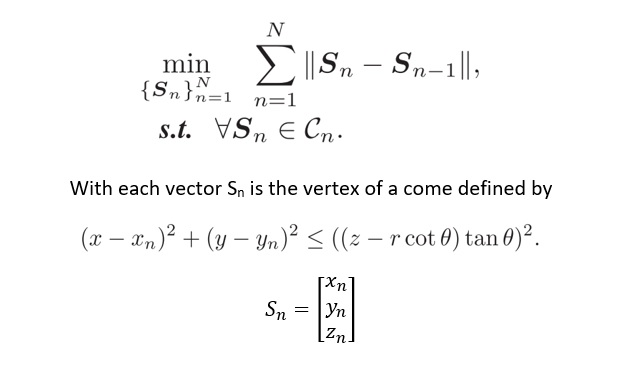# Shortest Distance between three vertices of cones

Greetings CVX Community:
My Optimization problem is as followI know that problem is CONVEX, I am not able to formulate the problem in CVX.
Can anybody help?

How do you know the constraint is convex?

It is a canonical region ( Cones ).

r and theta are constants

If it were just `z^2` on the RHS with `z >= 0`, this could be entered as `norm([x-xn y-yn]) <= z ` .If the values of `r` and `theta` are such that you are willing to impose
`(z-r*cot(theta))*tan(theta)` >= 0, then you are all set (or always <= 0, in which case reverse the sign of the LHS); otherwise, not.